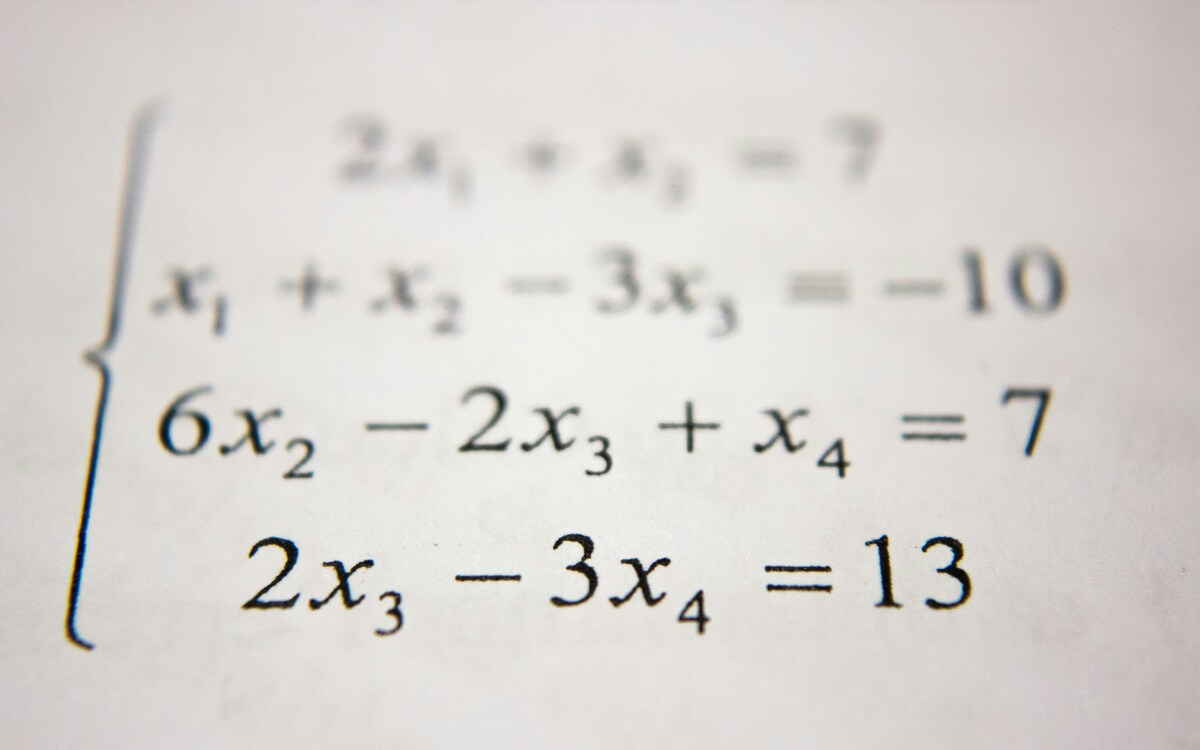# Ratio to Fraction Calculator

Converting the ratio to fraction is easy by using Ratio to Fraction Calculator. Not only converts ratio into a fraction but also reduces the fraction to its simplest form. Just enter the values in the input fields and hit the calculate button then you will get the answer in a split second.

Ratio to Fraction of:

Here are some samples of Ratio to Fraction calculations.

Related Calculators:

Ratio to Fraction Calculator: Do you want to convert ratio to fraction? The Ratio to Fraction Calculator will helps you to calculate ratios to fractions easily.

This tool works whether the ratios are comparing two numbers as a part or comparing a number as a whole. We have also share you definitions, example problems and explained every thing in detail.

## What is Ratio to Fraction?

In Maths, ratio to fraction is nothing but converting ratio into the simplified fraction form. There is a close link to the fraction and ratio. We can convey ratio to a fraction in 2 different ways. One is part-to-part ratio and another one is part-to-whole ratio. We will see one by one.

Part-to-Whole Ratio

Part-to-whole ratio is the relationship between the subset and the entire set. To convert ratio to fraction in the part-to-whole ratio, simply need to rewrite the ratio to fraction. For example ratio is a:b, and it is converted to a/b directly.

Part-to-Part Ratio

Part-to-part ratio is a subset of a set. If you want to convert ratio to fraction in part-to-part ratio, as the name itself says part-to-part, it will be converted to 2 different fractions. First, we will add both the numbers in the ratio, and then we will take that value as a denominator and then, each part of the ratio will become a fraction.

For example, if we take ratio as a:b then a+b = denominator(d), then we write as a/d and b/d and we will simplify them.

### Steps to Simplify Ratio to Fraction

As we have seen the above definition, here we will discuss the step by step process to convert ratio to fraction. As we can convert ratio to fraction in 2 methods we will see both of them.

Method1: Steps for Part-to-whole ratio to fraction

• First, you need to see the ratio that has given in the problem.
• Next, change the same given ratio into the fraction.
• Then the fraction will be changed to its simplest form. Like wise part-to-whole ratio will convert to simplified fraction.

Method2: Steps for Part-to-part ratio to fraction

• First, you need to see the ratio that has given in the problem.
• Next, add the two parts of the ratio, we will consider that answer as denominator.
• Then, we will place that denominator below both the parts of the ratio to get fractions.
• So, in this part-to-part ratio we will get two fractions.
• At last, those two fractions will be simplified and it will be changed to simple fraction.

### How to Convert Ratio to Fraction with Examples?

As we have 2 methods to convert Ratio to Fraction, Here we are going to show you examples for both the methods to understand easily.

Example for Part-to-Whole Ratio to Fraction

Question: Convert ratio 5:4 to fraction form?

Solution:

Ratio 5:4 can be converted in the fraction form as 5/4.

Example for Part-to-Part Ratio to Fraction

Question: Convert the ratio of 5 mangoes to 8 custurd apples in a fruit basket?

Solution:

Firstly, find the denominator by adding the parts i.e. 5+8 = 13

Now, put each part of the ratio above the denominator to get fractions.

Mangoes = 5/13

Custurd Apples = 8/13

Thus, in that fruit basket we have 5/13 of Mangoes and 8/13 of custurd apples.

### How to Use the Ratio to Fraction Calculator?

In this, you can use our calculator to convert the ratio to fraction. This calculator will give you both the methods.

• Firstly, give the numbers in the input fields which you can see.
• Next, click on the calculate button to get the answer.
• Then you will see the answer in no time along with the detailed explanation.

If you need any help in maths, physics, chemistry formulas and if you want to save time you can use our free calculator tools at multiplyfractions.com

### Frequently Asked Questions on Ratio to Fraction Conversion

1. How to convert ratio to fraction?

Ratio to fraction can be converted by using two simple methods.

2. What are the two methods to convert Ratio to fraction form?

The two methods to convert ratio to fraction is part-to-whole ratio and part-to-part ratio.

3. What is the ratio of 6:8 in the fraction form?

Ratio 6:8 can be expressed in fractions form as 6/8 and its simple form is 3/4.

4. How do I convert ratios to fractions easily?

you can use our ratio to fraction calculator in multiplyfractions.com to convert ratio to fraction easily and quickly.